# Team:Bielefeld-CeBiTec/Software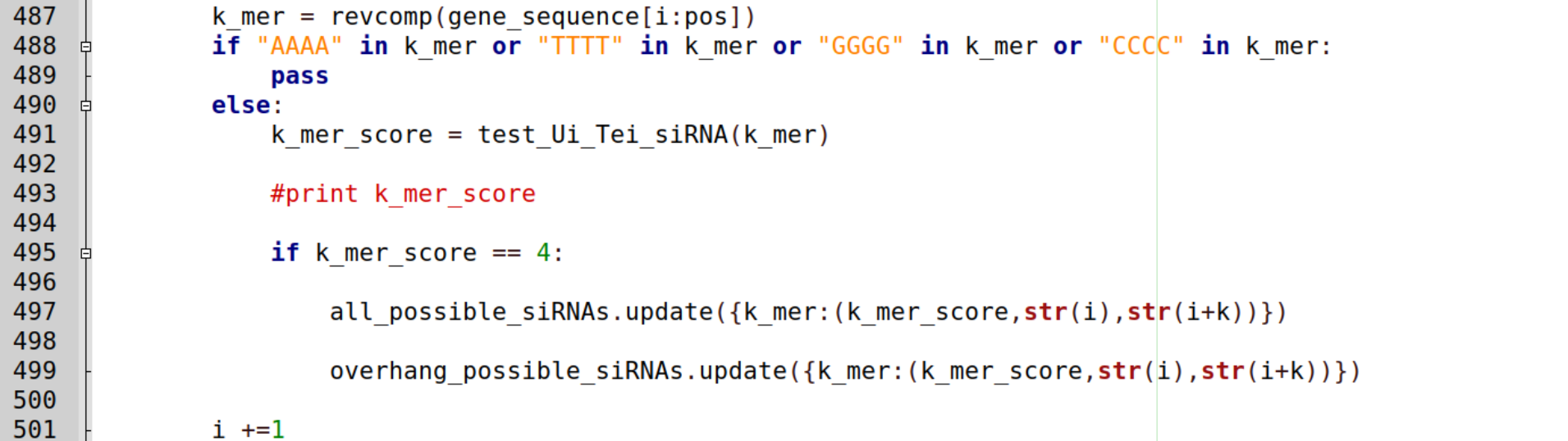siRCon - A siRNA Constructor

## Short Summary

In our project, we introduced RNA interference (RNAi) and translation repression with small interfering RNAs (siRNAs) as an alternative to CRISPR/Cas. To use siRNA as silencing agents for the gene-of -interest, we proposed a two-step design process. At first, potential siRNAs for prokaryotic organisms must be designed. In the second step, the silencing effect of these siRNAs can be validated by our siRNA vector system TACE. To facilitate the initial siRNA design step, we developed a siRNA construction tool which identifies possible siRNAs for a given gene sequence, calculates their probability to silence the target gene, and returns candidates ranked based on the calculated score. It consists of three modules: "siRNAs for RNAi", "siRNA", and "check siRNA". The siRNAs predicted by our software are perfectly compatible with our siRNA vector system. To the best of our knowledge, this is the first tool dedicated to predicting customized siRNA for application in prokaryotes. This Python tool comes in two versions: a command line application and an easy-to-use graphical interface.

## siRNAs short introduction

siRNAs are small, non-coding single-stranded RNAs with an average length of 21-25 nucleotides which bind a specific complementary coding mRNA and silence its function. In eukaryotic RNAi, siRNAs are loaded to Argonaute proteins which carry out the repression, either by blocking mRNA translation or by degrading the mRNA (Siomi and Siomi, 2009). More detailed information on both possible siRNAs mechanisms is found here.

## siRNA design

In order to achieve effective gene silencing or knock-down, the 19 nt binding sequence must be flanked by special, non-binding 5' and 3' extensions (Figure 1). To trigger mRNA degradation by RNase E, the 5’-terminal triphosphate of the siRNA needs to be converted to a monophosphate by RNA pyrophosphohydrolase (RppH). For the siRNA to be recognized by RppH, the 5’ end of the siRNA has to start with the tetranucleotide AGNN which is not allowed to match the targeted mRNA (Foley et al., 2015). At the 3’ end of the siRNA, the small MicC scaffold is added which facilitates the hybridization of siRNA and target mRNA and protects the siRNA from degradation (Na et al., 2013).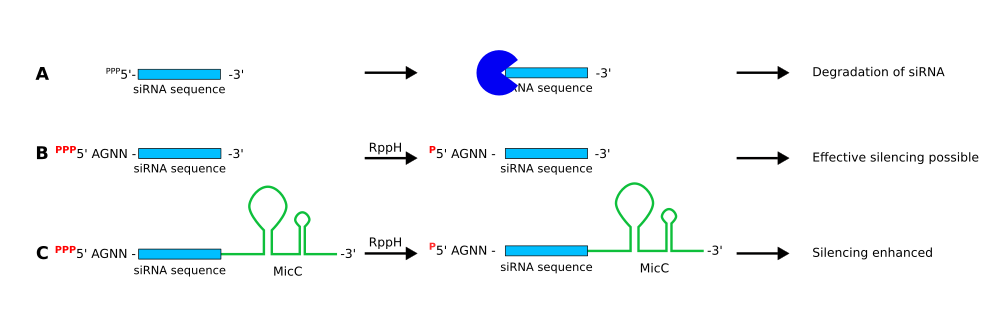Figure 1: Effects of siRNA design on RNAi effectiveness and siRNA stability. A If the siRNA does not carry suitable 5' or 3' extensions, it is quickly degraded. B siRNAs extended by the tetranucleotide AGNN are recognized and processed by the pyrophosphohydrolase RppH. This enzyme converts the 5' triphosphate to a monophosphate which greatly reduces siRNA degradation. This allows the siRNA to hybridize to its target mRNA which in turn is degraded by RNAse E, thus leading to effective mRNA silencing. C Extending siRNAs with a 3' MicC scaffold in addition to the 5' tetranucleotide AGNN further enhances mRNA silencing. MicC facilitates the hybridization of siRNA and target mRNA and protects the siRNA from degradation.
In addition to degradation-based RNAi, siRNA can also be used to block mRNAs without degradation. This is achieved by adding the outer membrane protein A (OmpA) scaffold to the 5' end of the siRNA (Figure 2), enhancing its stability. In addition, the hybridization of the siRNA and the target mRNA can be facilitated by addition of MicC to the 3' terminus.
Both sequence extensions are also part of our vector system, enabling efficient design and construction of effective siRNAs. If our vector system is selected when using our tool, the fitting overlaps to our vectors are added automatically. More theoretical information about the overhangs and scaffolds can be found here.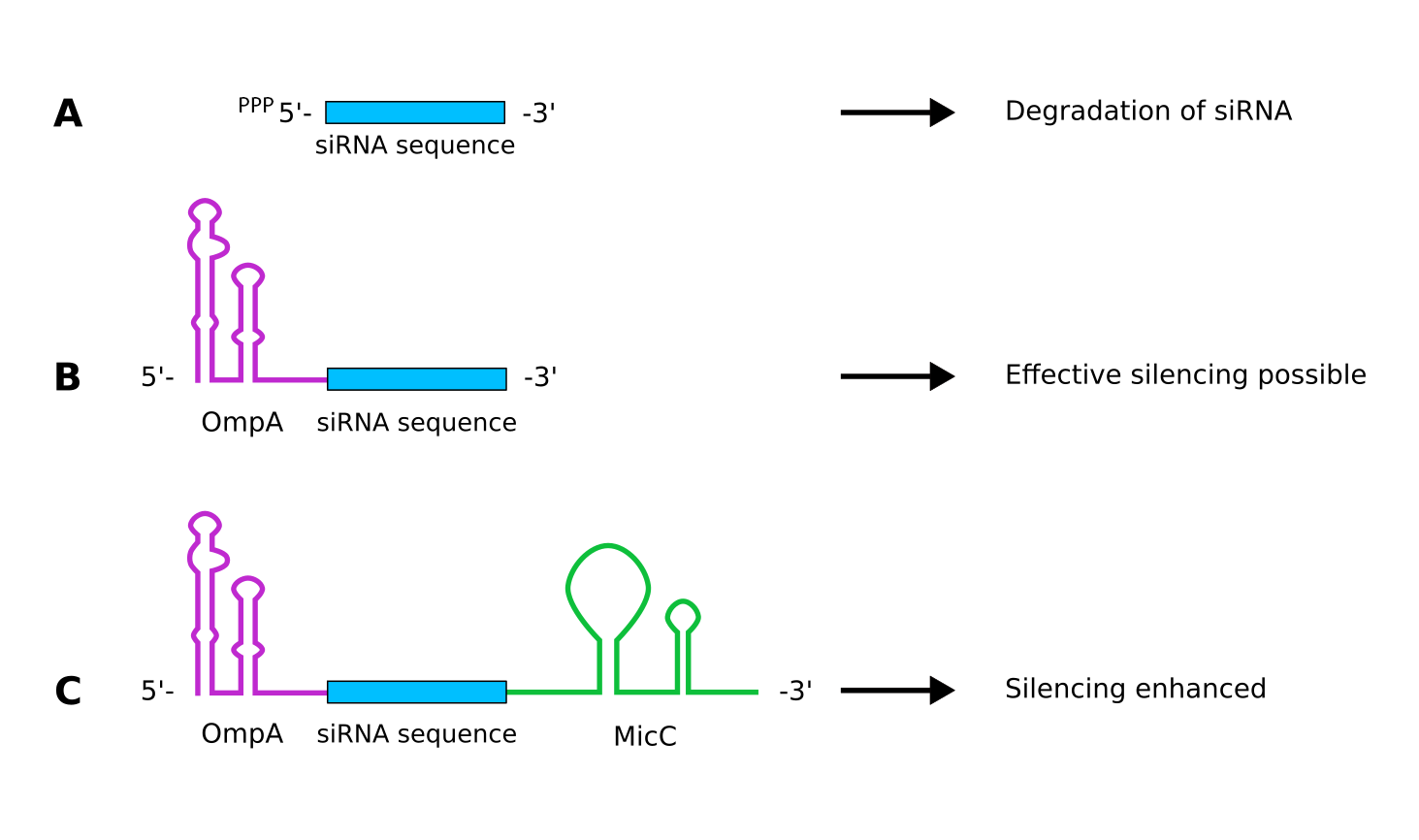Figure 2: siRNA design for silencing translation. A If the siRNA does not carry suitable 5' or 3' extensions, it is quickly degraded. B siRNAs supplemented with the outer membrane protein A (OmpA) scaffold are more stable and effectively silence the translation of target mRNAs. C If the siRNA is supplemented with the OmpA as well as the MicC scaffold the repression is enhanced further.

## Choosing appropriate design methods

In 2012, the iGEM team SYSU-Software integrated an siRNA cDNA designer as a small part of their project. siRNAs designed with this tool were applicable in eukaryotic organisms. They included two different design methods: Tom Tuschl’s method and Rational siRNA design.
In the following as well as in our software tool siRCon, nucleotide sequences exclusively contain the letter 'T' for sake of simplicity. Please note that in the case of RNA, the corresponding base is uracil.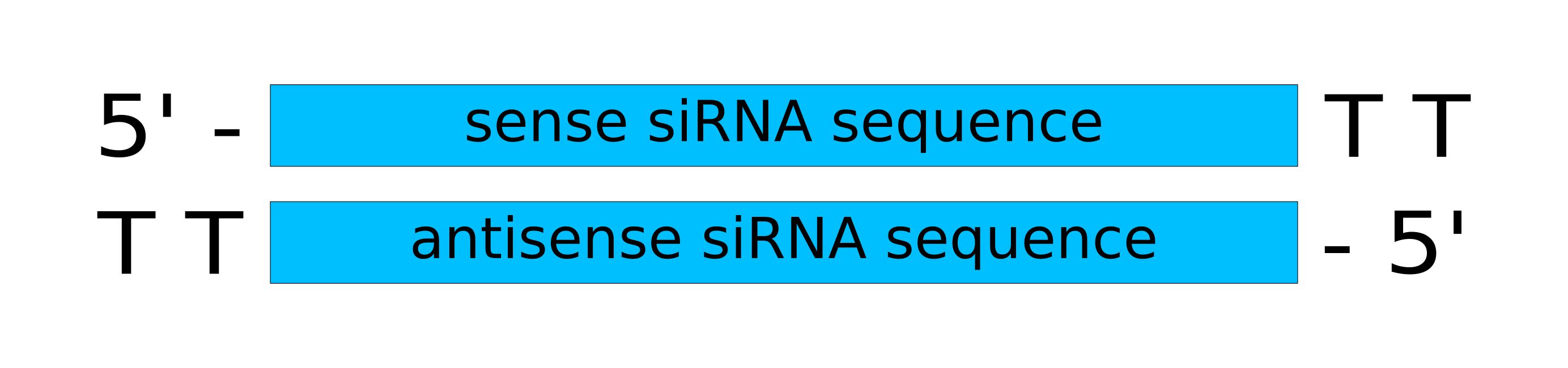Figure 3: Structure of an siRNA designed with Tom Tuschl's method. Both siRNA have a characteristic 'TT' overhang at the 3'-terminus (Elbashir et al., 2001).
Tom Tuschl’s method focuses mainly on the existence of 5’ and 3’ ‘TT’ overhangs (Figure 3) (Elbashir et al., 2001). These are not compatible with overhangs and scaffold sequences required by the prokaryotic mechanisms. Therefore, we decided to use the rules published by Ui-Tei as an alternative design method (Naito and Ui-Tei, 2012). Furthermore, we adapted the rational siRNA design as it was more suitable for our application (Reynolds et al., 2004). Both design rules apply only to the 19 nt long target binding sequence.

## Rational siRNA design

By a systematic analysis of 180 eukaryotic siRNAs, Reynolds et al. identified eight criteria that are important for their functionality (Reynolds et al., 2004). Each criterion gets a score that is either positive or negative, corresponding to its effect on the siRNA. All siRNA candidates with a score above six are potential highly functional siRNAs.
Table 1:Rational siRNA design criteria with corresponding score (Reynolds et al., 2004)
Rule Score
30%-52% G/C content +1
At least 3 'W' ('A' or 'T') at positions 15-19 +1 (for each 'A' or 'T')
Absence of internal repeats ($$T_m \lt 20$$) +1
An 'A' at position 3 +1
An 'A' at position 19 +1
A 'T' at position 19 +1
An 'A' or 'T' at position 19 -1
An 'A', 'C' or 'T' at position 13 -1
The melting temperature Tm is calculated as follows (Kibbe, 2007): $$T_m = 79.8 + (18.5 * log_{10}[Na^+]) + (58.4 * [\text{G/C content}]) \\+ (11.8 * [\text{G/C content}]^2) - \left(\frac{820}{\text{[G/C content]}}\right)$$

## Ui-Tei rule

Ui-Tei et al. analyzed 62 eukaryotic siRNAs and identified four design rules for effective siRNAs (Ui-Tei, 2004). Only siRNAs fulfilling all four criteria are considered functional siRNAs.
1. An ‘A’ or ‘T’ at position 19
2. A ‘G’ or ‘C’ at position 1
3. At least five ‘T’ or ‘A’ residues from positions 13 to 19
4. No ‘GC’ stretch more than 9 nt long

## Calculating silencing probability

Our software siRCon should report not only the sequences of potential effective siRNAs, but also rank them based on the probability with which they are effective. This is calculated with the help of Bayes’ theorem by calculating probabilities of dependent events. The following calculations and formulas are based on Takasaki (2009).
TThe initial hypothesis is that the given siRNA effectively silences an mRNA. To perform the calculations, a prior probability is necessary. The prior probability for effective gene silencing of mammalian genes can be obtained from former siRNA experiments and is approximately 0.1 (Takasaki, 2009). Since we have no data on prokaryotic siRNAs, we use the same prior probability for our predictions.
The gene silencing probability $$P(eff|X)$$ is described as: $$P(eff|X) = \frac{P^{eff} P(X|eff)}{P^{eff} P(X|eff) + P^{inf} P(X|inf)} \qquad (1)$$ The 19 nt siRNA binding sequence is represented by X, where $$x_i^n$$ corresponds to the bases adenine, guanine, cytosine or uracil (indexes 1≤n≤4) at sequence position i. The probabilities P(X|eff) and P(X|inf) are calculated based on prior knowledge about siRNA sequences that were shown to be effective respectively ineffective in silencing their target mRNAs. Based on the analysis of 833 effective and 847 ineffective siRNAs, Takasaki et al. determined the likelyhood with which base n occures at position i in an effective/ineffective siRNA sequences, represented by the coefficients $$q_{x_i^n}^{eff}$$ and $$q_{x_i^n}^{inf}$$ respectively (Takasaki, 2009). These coeffecients are often referred to as frequency ratios of n at position i.
$$P(X|eff)$$ and $$P(X|inf)$$ are computed as the product of the frequency ratios for each base n at position i in the siRNA binding sequence: $$P(X|inf) = \prod_{i=1}^{19} q_{x_i^n}^{eff} \qquad (2)$$ $$P(X|inf) = \prod_{i=1}^{19} q_{x_i^n}^{inf} \qquad (3)$$
Both probabilities are weighted with their prior probabilities, $$P^{eff}$$ and $$P^{inf} = 1-P^{eff}$$, where $$P^{eff}$$ is set to 0.1 as mentioned previously. With all defined formulas (1), (2) and (3), the gene silencing probability $$P(eff|X)$$ is calculated as follows: $$P(eff|X) = \frac{P^{eff} P(X|eff)}{P^{eff} P(X|eff)+P^{inf} P(X|inf)} \\\\= \frac{P^{eff} \prod_{i=1}^{19} q_{x_i^n}^{eff}}{P^{eff} \prod_{i=1}^{19} q_{x_i^n}^{eff}+P^{inf} \prod_{i=1}^{19} q_{x_i^n}^{inf}}$$
In order to actually calculate the silencing probability, only the frequency ratios $$q_{x_i^n}^{eff}$$ and $$q_{x_i^n}^{inf}$$ of the individual nucleotides at positions 1 to 19 are missing. These could be taken from the same publication from Takasaki as the calculations (Takasaki, 2009).
For each nucleotide, the probability of occurrence was determined for each position of the siRNA. Different models were taken into account in the calculation. First of all, the occurrence of the different nucleotides at positions 1 to 19 can be considered as independent of each other. The probabilities for each position are then calculated independently. However, the occurrences of the nucleotides can also be considered as dependent of each other. This means that the occurrence of a nucleotide depends on the nucleotide at the position before. For the calculation of dependent probabilities, the Simple Markow Model was used. It has been found that the resulting silence probability is most accurate when the frequency ratios of the effective siRNAs are calculated dependent and the frequency ratios of the ineffective siRNAs are calculated independent. All frequency ratios can be looked up here.
In combination with the frequency ratios it is now possible to calculate the silencing probability for the 19 bp long binding site of siRNAs.

## siRNA selection for RNAi and repression of translation

The procedures of siRNA selection for both mechanisms, RNAi and repression of translation, are very similar. Thus the first two modules, RNAi and siRNA, are similar. First the mRNA binding sequence is determined using the rational design and the Ui-Tei rules. In the next step, the silencing probability is determined. At the end, the corresponding overhangs and scaffolds are added to the 19 nt long binding sequence to form the mature siRNA.

## Check siRNA

Beside the selection of siRNAs, we also implemented a functionality to check siRNAs derived by other methods. For a given target sequence and a corresponding siRNA it is checked whether the siRNA might bind to its target and how well it fulfills the described criteria. Furthermore, its silencing efficiency is calculated.

## Command line application

The command line application can be obtained directly here or downloaded from our GitHub repository. To run the command line application, Python 2.7 needs to be installed.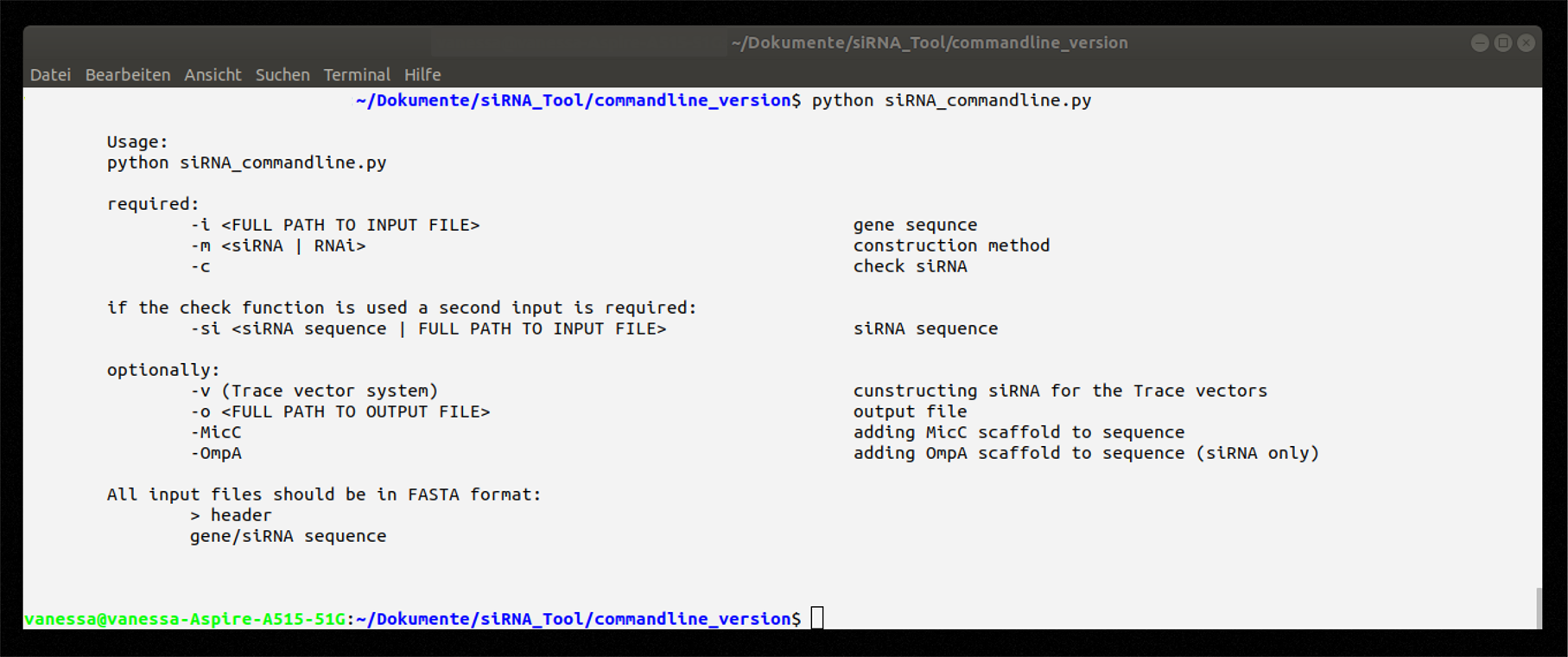Figure 4: Help message on how to use the command line application.
Used without input, a help message is displayed listing the mandatory and optional input parameters (Figure 4). For more information a README is available in our repository. All resulting siRNAs are saved in one FASTA file. This simplifies the integration into different workflows. For example, it is possible to test the siRNAs on off-target bindings site using BLAST. An exemplary call of the application as well as the results returned can be seen in Figure 5.Figure 5: Exemplary call and results of the command line application using a GFP gene sequence as input.

## Graphical Interface usage

Like the command line application, the graphical interface version can either be downloaded directly here, or via our GitHub repository. In the graphical interface, the modules are accessible via tabs (Figure 6). The last tab contains usage and copyright information.

### Tab 1: siRNA for RNAi

1. Insert gene sequence
2. Choose TACE vector system (optionally)
3. Constructions of siRNAs
4. View resulting siRNAs (sense and antisense sequence) and their corresponding probability
5. Decide if siRNAs should be saved with MicC scaffold (only if TACE is not used)
6. Save results as FASTA file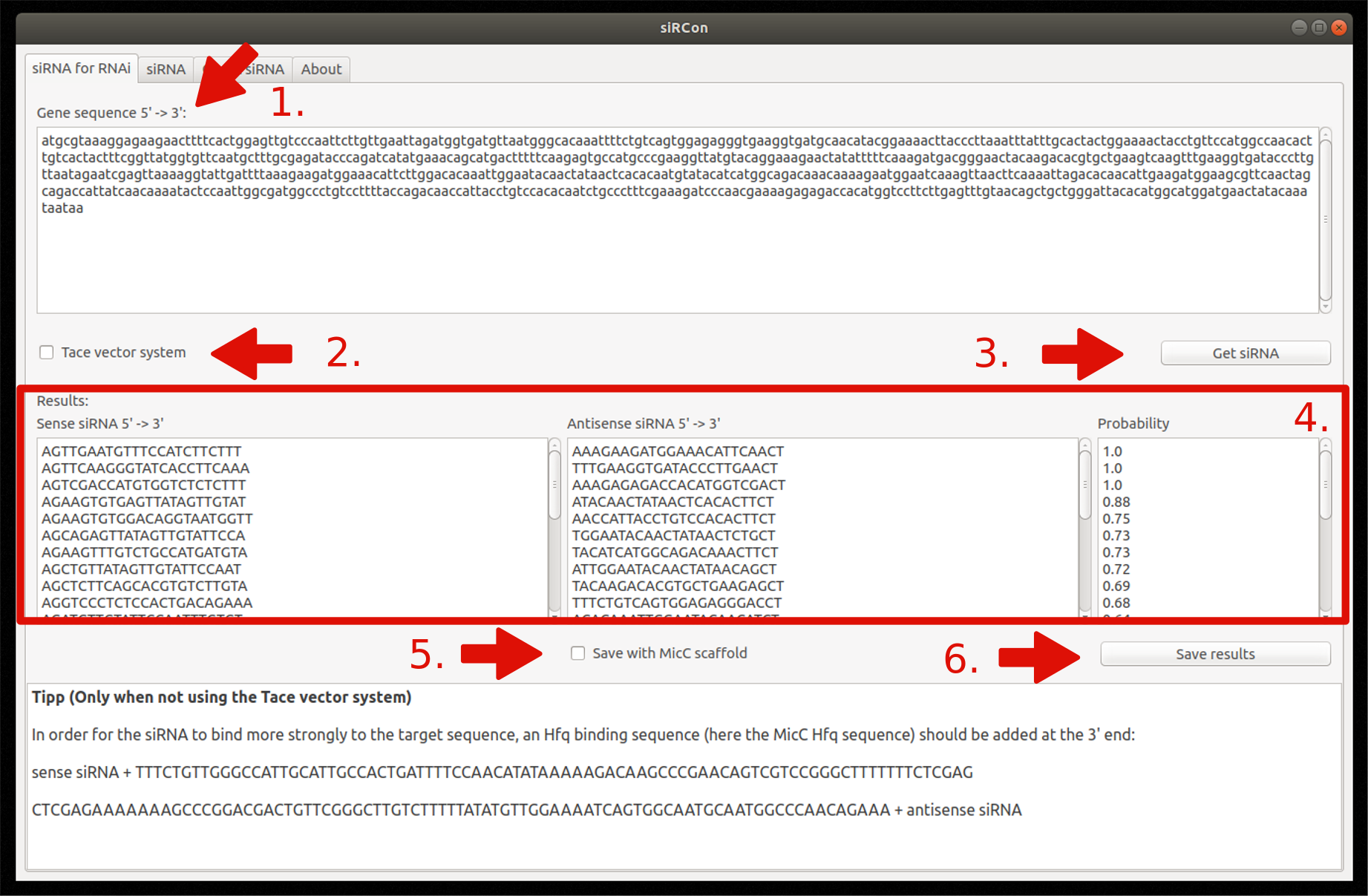Figure 7: Overview and steps of the siRNA for RNAi module.

### Tab 2: siRNA for silencing

1. Insert gene sequence
2. Choose TACE vector system (optionally)
3. Constructions of siRNAs
4. View resulting siRNAs (sense and antisense sequence) and their corresponding probability
5. Decide if siRNAs should be saved with MicC scaffold (only if TACE is not used)
6. Decide if siRNAs should be saved with OmpA scaffold (only if TACE is not used)
7. Save results as FASTA file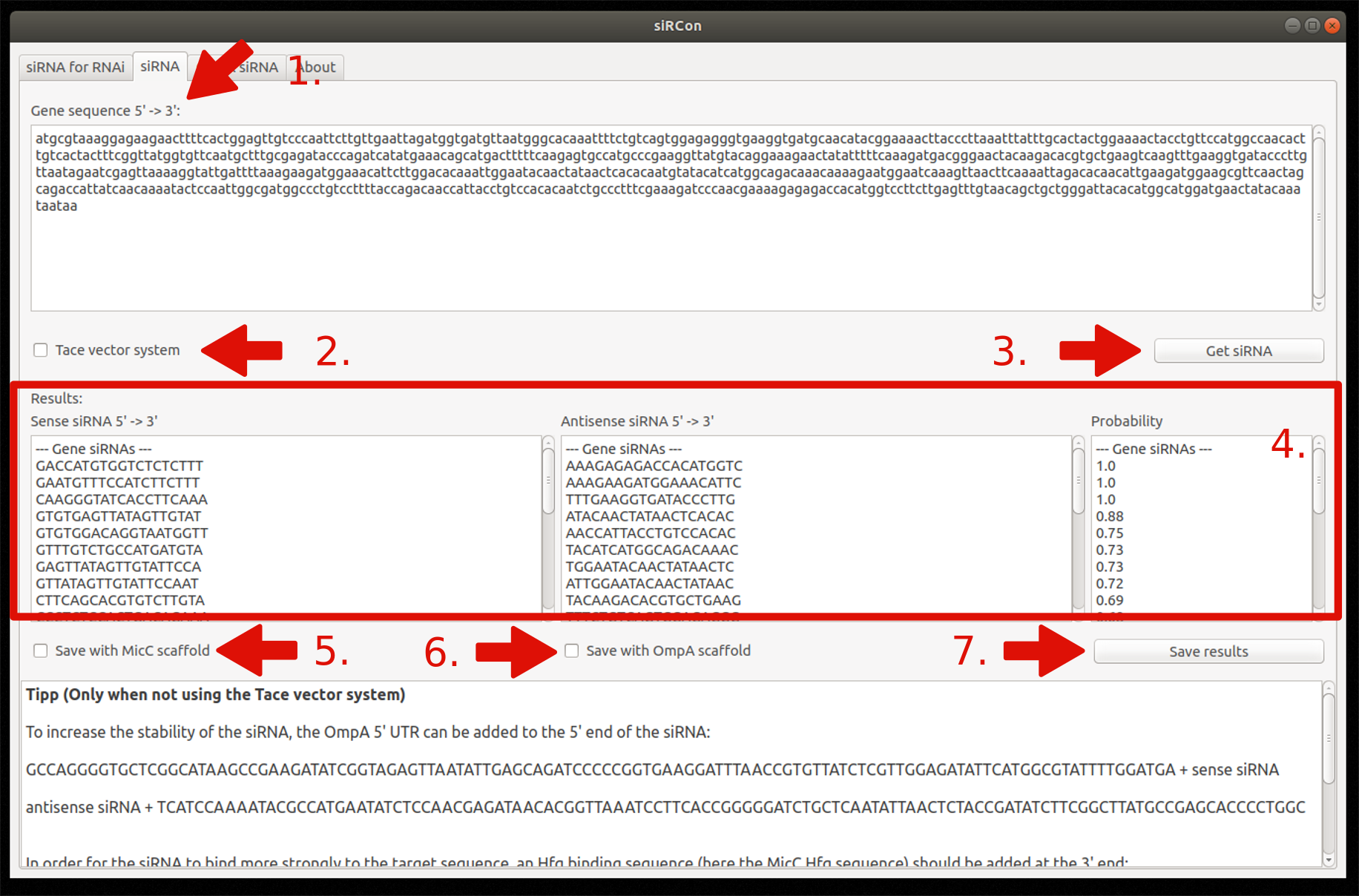Figure 8: Overview and steps of the siRNA for silencing module.

## Tab 3: Check siRNA

1. Insert gene sequence
2. Insert siRNA sequences
3. Choose method the siRNA was constructed for (siRNA for RNAi or siRNA for silencing)
4. Choose if siRNA was constructed for TACE (optionally)
5. Validation of entered siRNA for given target gene sequences
6. View results
7. Save results (optionally)

## Outlook

To help future iGEM teams to control gene expression, we developed siRCon, a bioinformatic application to generate high-fidelity siRNA sequences in prokaryotic organisms. We introduce this method as an alternative to CRISPR/Cas, since it is open source and free of charge. In the future, further improvements and extensions of this applications are intended. On the one side, eukaryotic siRNAs will also be constructed. This is how we want to provide a universal tool for siRNAs. On the other side, we want to improve the already existing features, especially the check siRNA functionality.
Elbashir, S.M., Harborth, J., Lendeckel, W., Yalcin, A., Weber, K., and Tuschl, T. (2001). Duplexes of 21-nucleotide RNAs mediate RNA interference in cultured mammalian cells. Nature 411: 494–498.
Foley, P.L., Hsieh, P., Luciano, D.J., and Belasco, J.G. (2015). Specificity and evolutionary conservation of the Escherichia coli RNA pyrophosphohydrolase RppH. J. Biol. Chem. 290: 9478–9486.
Kibbe, W.A. (2007). OligoCalc: an online oligonucleotide properties calculator. Nucleic Acids Res 35: W43–W46.
Na, D., Yoo, S.M., Chung, H., Park, H., Park, J.H., and Lee, S.Y. (2013). Metabolic engineering of Escherichia coli using synthetic small regulatory RNAs. Nat. Biotechnol. 31: 170–174.
Naito, Y. and Ui-Tei, K. (2012). siRNA Design Software for a Target Gene-Specific RNA Interference. Front Genet 3.
Reynolds, A., Leake, D., Boese, Q., Scaringe, S., Marshall, W.S., and Khvorova, A. (2004). Rational siRNA design for RNA interference. Nature Biotechnology 22: 326–330.
Siomi, H. and Siomi, M.C. (2009). On the road to reading the RNA-interference code. Nature 457: 396–404.
Takasaki, S. (2009). Selecting effective siRNA target sequences by using Bayes’ theorem. Computational Biology and Chemistry 33: 368–372.
Ui-Tei, K., Naito, Y., Takahashi, F., Haraguchi, T., Ohki-Hamazaki, H., Juni, A., Ueda, R. and Saigo, K. (2004). Guidelines for the selection of highly effective siRNA sequences for mammalian and chick RNA interference. Nucleic Acids Res. 32: 936-948.